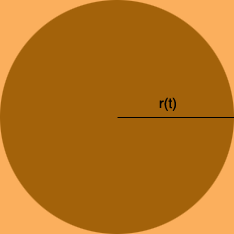SEARCH HOMEMath Central Quandaries & QueriesQuestion from Amanda, a student: A circle is inscribed in a square. The circumference of the circle is increasing at a rate of 6 inches per second. As the circle expands, the square expands to maintain the tangency. Determine the rate at which the area of the region between the circle and square is changing at the moment when the circle has an area of 25(pi) square inches. Please help. Thank you.Hi Amanda,

The radius of the circle is not fixed, it changes with time so to emphasize this I am going to cal the radius of the circle r(t) inches where t is time measured in seconds.What is the length of a side of the square?

What is the area of the square?

What is the area of the circle?

Write an expression for A(t), the area of the region between the circle and the square,

A(t) = (area of the square) - (area of the circle).

Simplify.

Now it's time to use the calculus you know. Differentiate both sides with respect to t. This will result is A'(t) on the left side and an expression involving r'(t) on the right. You know the value of r'(t), it's 6 inches per second. What is the radius of the circle when the area of the circle is 25 π square inches? What is A'(t) at this time?

I hope this helps,
PennyMath Central is supported by the University of Regina and The Pacific Institute for the Mathematical Sciences.